# Venn Diagram

• Updated on

## What is a Venn Diagram?

A Venn diagram is a graphical representation that shows the relationship between a finite group of things. In a Venn diagram, the relationship between the group of things, called sets, is explained with the help of circles.

In case there is something common between two sets of data, then the circles would overlap. On the other hand, if the two sets of data are distinct, the circles will not overlap.

Let us take an example to understand the concept:

Suppose A is a set of numbers that are divisible by 2 and B is a set of numbers that are divisible by 3. In these two sets, there would be some common numbers as well, which are divisible by both 2 and 3.

A= {2, 4, 6, 8,10, 12, 14, 16, 18, 20}

B= {3,6,9,12,15,18,21,24,27,30}

Graphically the above statement is interpreted using a Venn Diagram as below: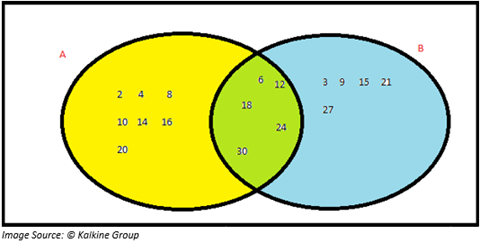The yellow region represents numbers that are only divisible by 2 while blue represents those numbers that are divisible 3 only. The section highlighted in green describes the numbers that are multiples of both 2 and 3.

Mathematically,

• (A U B), or A union B, comprises all the numbers from sets A and B.

(A U B) = {2,3,4,6,8,9,10,12,15,16,18,20,21,24,27,30}

• (A ∩ B), or A intersection B, represents the numbers common for both sets A and B.

(A ∩ B) = {6,12,18,24,30}

## Sets and Venn Diagrams:

A set is defined as a list of elements. In other words, it is the grouping of individual elements into a whole. It is essential to understand that the elements are clear and unambiguous.

An example of an unclear set: {set of tall boys}

An example of an unambiguous set: {set of even numbers less than 20}

## What are the different types of sets?

1. Singleton set: Singleton set has only one element within the set.
2. Finite set: Finite set has a limited number of objects. E.g., set of numbers divisible by 2 and less than 20.
3. Infinite set: A set of rational numbers between 0 and 1. This is an example of an infinite set.
4. Equal set: If two sets have the same elements, then we say that two sets are equal.
5. Null or Empty set: Null or empty set has no element.
6. Subset: If we say that ‘A’ is a subset of ‘B’, then it means that each element of set A is there in set B. The subset is represented using symbol “?”.
7. Proper set: Set A is a proper subset of B if A is a subset of B and A is not equal to B. It can also be described as A is a subset of B; however, B has at least one such element which is not a part of A.
8. Improper set: In improper subset, A is said to be an improper subset of B if A=B.
9. Power set: Power set refers to all possible combination of sets. E.g.: A={1,2}, then P(A)= {{}.{1}, {2},{1,2})
10. Universal set: Universal set is the superset of all the subsets under consideration.

## How do Venn Diagrams help users?

As described above, a set describes a group of elements which are represented within curly brackets ({, }). Now suppose there are three sets, and we want to understand if there exists a relationship between these sets. In such a scenario, Venn diagrams prove useful.

Now, the question which arises here is that how we can establish a relationship between these sets. The relationship between the sets can be understood through various symbols, as discussed below.

To understand the relationship, let us consider an example where there are two sets of players from cricket and football. Students is the set of all students studying in a class (universal set).

Students = {A, B, C, D, E, F, G, H, I, J, K, L,M}

Cricket = {A, B, C, D, E, F}

Football= {E, F, G, H, I, J}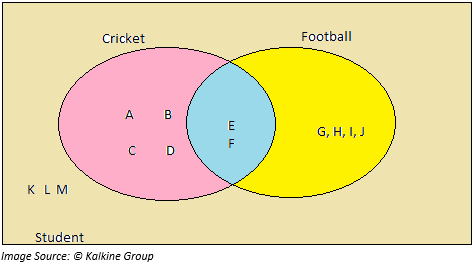Union (?)

In Union, the elements of two or more sets are combined. Now, if we want to know the name of all those students playing cricket or football or both, then we use union. So, we represent that the set of students playing cricket or football, or both are:

Cricket ? Football = {A, B, C, D, E, F, G, H, I, J}

Through the Venn diagram, “Cricket ? Football” includes those students that either play cricket or football or both (area highlighted in pink).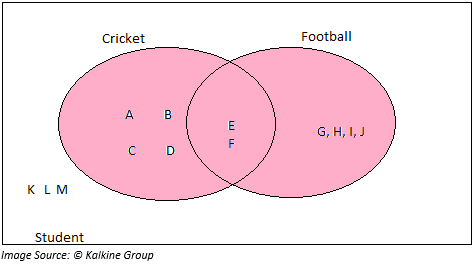Intersection (∩):

In an intersection, the set comprises of these elements which are common in both the sets. In this case, two students are playing both cricket and football.

Cricket ∩ Football = {E, F}

Through the Venn diagram, “Cricket ∩ Football” includes those students highlighted in the pink region.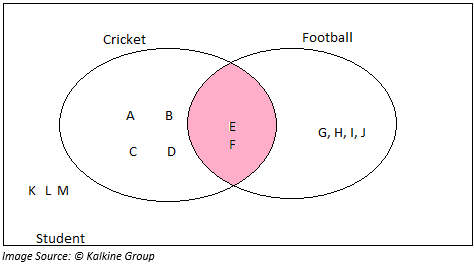Difference (-):

In simple terms, a difference in mathematics means to subtract. So, if we say Cricket – Football, then the set would include only those players who are playing cricket and not football.

Cricket – Football = {A, B, C, D}

Through the Venn diagram, “Cricket – Football” includes those students highlighted in the pink region.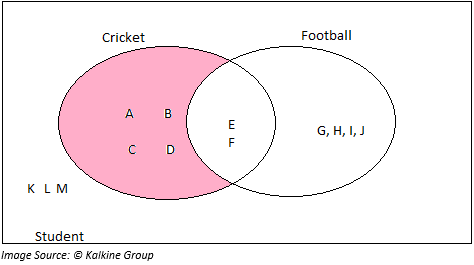Compliment (S’ or Sc, where S is set):

If we say Footballc or Football compliment, it means that the set would include all the students in the classroom who are not playing football at all.

In this case,

Footballc = {A, B, C, D, K, L,M}

Through the Venn diagram, “ Footballc “  includes those students highlighted in the pink region.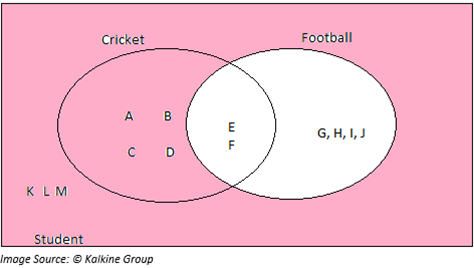## Applications of Venn Diagram:

Some cases where Venn Diagrams can be useful include:

• Observing a Student's Behaviour
• Understanding the interaction of the students in the class
• Marketing analysis
• Tracking the health of the patient
• Support in identifying the respective responsibilities of state and federal government

##### Top ASX Listed Companies

Definition:
We use cookies to ensure that we give you the best experience on our website. If you continue to use this site we will assume that you are happy with it. OK